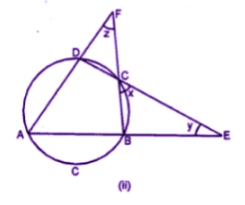Guru

# Question 8. (a) In the figure (i) given below, AB is a diameter of the circle. If ∠ADC = 120°, find ∠CAB. (b) In the figure (ii) given below, sides AB and DC of a cyclic quadrilateral ABCD are produced to meet at E, the sides AD and BC are produced to meet at F. If x : y : z = 3 : 4 : 5, find the values of x, y and z.

• 0

This is an important and exam oriented question from Chapter name- circles
Topic – Angle properties of circles
Chapter number- 15

In first qies AB is a diameter of the circle. If ∠ADC = 120°, we have to find ∠CAB.
In the figure (ii) , sides AB and DC of a cyclic quadrilateral ABCD are produced to meet at E, the sides AD and BC are produced to meet at F.   x : y : z = 3 : 4 : 5, now we have to find the values of x, y and z.
ICSE Avichal publication
Understanding ICSE Mathematics
Question 8

Share

1. Solution:

(a) Construction: Join BC, and AC thenNow in ∆DCF

Ext. ∠2 = x + z

and in ∆CBE

Ext. ∠1 = x + y

x + y + x + z = ∠1 + ∠2

2 x + y + z = 180o